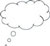# 4 The division algorithm again

The next activity returns to considering the formal division algorithm. It is framed to ask the students to start to formulate the division algorithm for themselves.

Activities 1 and 2 have asked the students to visualise what is happening when they use division and also to start to talk about remainders. Activity 3 begins the process of formalising these ideas into the kind of mathematical language that can be found in mathematical textbooks. The activity also starts the process, (continued in Activity 4), of asking the students to write mathematics as mathematicians do.

## Activity 3: The division algorithm

Rajni finally decides to use the Pink Sparrow tiles for her floor.

• How many complete tiles will be used along the length of the floor? What is the total length covered by these tiles? How much is the length of the floor that remains uncovered after the maximum number of tiles have been put along the length?
• Let q = number of complete tiles used along the length of the room.
• Let r = the length of the room that remains uncovered after the maximum number of complete tiles have been put along the length.
• Write a relation between: length of room, length of one tile, q and r.
• Repeat the first step using the breadth of the floor.Video: Assessing progress and performance

## Case Study 3: Mrs Agarwal reflects on using Activity 3

The students had done the two previous activities where they had seen that the tiles could completely cover the area of the floor lengthwise, except for one small bit, therefore they could tell how much of the length was not covered by the tiles.

I told them that this activity was going to ask them to be mathematicians and to use symbols to represent what they were doing when they used division. This seemed to spur them on and they worked hard in their groups for a short time, quickly writing the relationship between these various quantities in symbols. I asked several students to come to the blackboard and write down the relationship that their group had decided on. There were some small differences but the class quickly agreed on the way that we, as mathematicians, should write the division algorithm for this situation.

After they had done the work on the lengthwise relationship, I asked them to work in the same way on the breadth and they came up with that relationship very quickly as well.

It was good to see that even the students who normally would not take part in maths activities were contributing to the whole exercise. I think they could relate to this context, visualising what was going on, much better than if I had just told them the division algorithm. When I told them what the relationship they had found was called, the division algorithm, they all felt very important writing mathematics as mathematicians and coming up with such a formal sounding relationship.

After that I asked them to use the division algorithm to verify their answers to the previous activities and they could all do it and were able to, I think, understand what division actually means.Pause for thought Mrs Agarwal commented that even students who normally were reluctant to take part in maths activities made a contribution. This is great! What approaches might she have used to find out for certain that they had understood the division algorithm, or whether they still needed further opportunities to consolidate their understanding? After reflecting on this, you may find it useful to have a look at the key resource ‘Assessing progress and performance’.

3 Making connections

5 Working on a bigger scale